# 极坐标与笛卡尔坐标

。。。。。。及其互相转换。

## 笛卡尔坐标系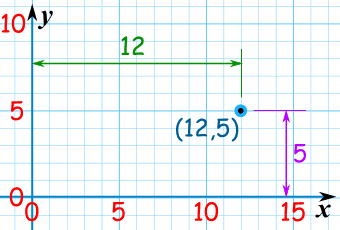## 极坐标系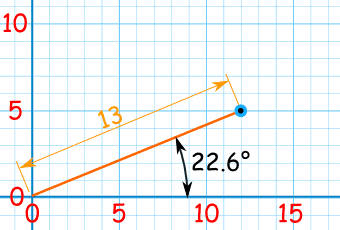## 转换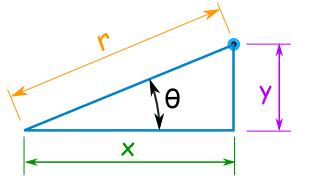## 笛卡尔坐标转换为极坐标

### 例子： (12,5) 的极坐标是什么？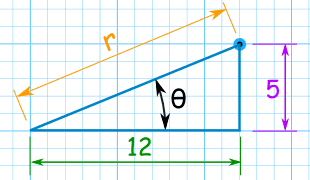r2 = 122 + 52
r = √ (122 + 52)
r = √ (144 + 25)
r = √ (169) = 13

tan( θ ) = 5 / 12
θ = tan-1 ( 5 / 12 ) = 22.6° （精确到一位小数）### 什么是 tan-1？

• 正切 已知一个角度为变量数得到一个比例，
• 反正切 已知一个比例 （像 "5/12"）得到一个角度。

### 所以，笛卡尔坐标 (x,y) 转换为极坐标 (r,θ):

• r = √ ( x2 + y2 )
• θ = tan-1 ( y / x )

## 极坐标转换为笛卡尔坐标

### 例子：(13, 22.6°)的笛卡尔坐标是什么？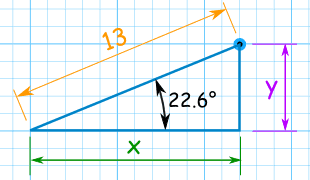用 x 的 余弦函数： cos( 22.6 °) = x / 13 重排及解： x = 13 × cos( 22.6 °) x = 13 × 0.923 x = 12.002... 用 y 的 正弦函数： sin( 22.6 °) = y / 13 重排及解： y = 13 × sin( 22.6 °) y = 13 × 0.391 y = 4.996……

• x = r × cos( θ )
• y = r × sin( θ )

## 但如果 X 和 Y 是负数呢？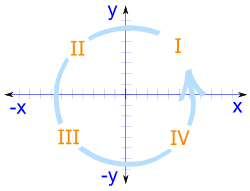4 个部分：

（逆时钟方向排列）

### 例子：(12, 195°) 的笛卡尔坐标是什么？

r = 12 和 θ = 195°

• x = 12 × cos(195°)
x = 12 × -0.9659...
x = −11.59 to 2 decimal places
• y = 12 × sin(195°)
y = 12 × -0.2588...
y = −3.11 精确到两位小数

。。。。。。计算器可能会为 tan-1

 象限 tan-1的值 I 用计算器的值 II 用计算器的值加上 180° III 用计算器的值加上 180° IV 用计算器上的值 加上 360°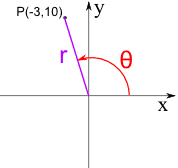### 例子：P = (−3, 10)

P 是在象限 II

• r = √((−3)2 + 102)
r = √109 = 10.4 精确到一位小数
• θ = tan-1(10/−3)
θ = tan-1(−3.33...)

tan-1(−3.33...) 的值是 −73.3°

θ 计算机得到的值= −73.3° + 180° = 106.7°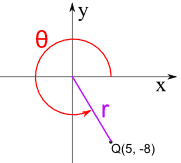### 例子：Q = (5, −8)

Q 是在 象限 IV

• r = √(52 + (−8)2)
r = √89 = 9.4 精确到一位小数
• θ = tan-1(−8/5)
θ = tan-1(−1.6)

θ = −58.0° + 360° = 302.0°

## 总结

• x = r × cos( θ )
• y = r × sin( θ )

• r = √ ( x2 + y2 )
• θ = tan-1 ( y / x )

tan-1( y/x ) 可能需要调整：

• 象限 I: 用 计算器的值
• 象限 II: 加 180°
• 象限 III: 加 180°
• 象限 IV: 加 360°